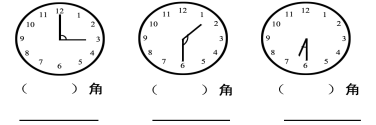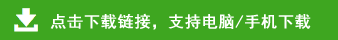# ​小学数学二年级下册易错题检测

一、填空。（14分，每空0.5分）

13084是由（　　）个千、（　　）个十和（　　）个一组成的
2. 在（    ）÷6=4……（    ），第二个括号最多只能填（    ）
3. 四千写作（      ） 3007读作（      ）
4. 790379309730973按从小到大的顺序排列：
（　 　）＜（　 　）＜（　　 ）＜（ 　　）
5.选择合适的单位填空（千米、米、分米、厘米、时、分钟、秒）

61km＝（　　）
1分＝（　　）秒
4000cm＝（　　）dm
7. 在计算35－35÷时 要先算（    ）法，再算（    ）法.
8. 一个五位数，它的最高位是（　　）位，最高位是百位的数是（　　）位数
9. 在○里填“＞”或“＜”
1003999　　　　　39684001　　　　　9002千克

10.下面各钟面上的时针和分针形成什么角？再写出钟面上的时刻。1在有余数的除法里余数一定要比除数小   （    ）
2锐角比直角大 （    ）
3四位数都比三位数大   （    ）
4学校的操场跑道约200（   ），括号里的单位应该是mm  （    ）
5一个角有一个顶点，两条边.     (     )

1直接写出下列各题的得数（6分，每小题0.5分）
303－170 =         28÷4 =
25 + 41 =            6×7 =
87－55 =           51÷7 =
3745 =            7126 =
1200－600 =        59002000 =

74 + 32 =            12050 =